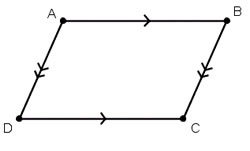# Difference between Trapezium and Parallelogram

##### Key Difference: A trapezium is a quadrilateral is that has at least one pair of parallel sides. The figure is more commonly known as a trapezoid. A parallelogram is a quadrilateral that has two pairs of parallel sides.The words trapezium and parallelogram are commonly found in mathematics and geometry. These terms refer to geometric shapes that are commonly used to learn and understand angles. Both, trapezium and parallelogram are quadrilaterals and may result in it being confusing for people that are not actively using geometry. These figures also play an important part in architecture.

A trapezium is a quadrilateral is that has at least one pair of parallel sides. The figure is popularly known as trapezoid in most parts of the world, but referred to as trapezium in certain countries such as the UK. According to Math Open reference the name suggests other differences. A trapezium in the United States refers to a quadrilateral with no parallel sides, while a trapezoid refers to a quadrilateral that has one pair of parallel sides. However, in the UK it is considered the opposite; a trapezoid is considered to be a quadrilateral with no parallel sides, while a trapezium is considered to a quadrilateral with one pair of parallel sides.

The parallel sides of a trapezium/trapezoid are called the bases of the trapezoid and the other two sides are called the legs or the lateral sides. However, if the lateral sides of legs are parallel then the trapezoid would have two bases. There is some disagreement to the actual definition of trapezium, with some saying that a trapezium has exactly one pair of parallel sides, while others define a trapezium having at least one pair of parallel sides. According to the former definition, a parallelogram would not be considered as a trapezium, while the latter definition states that a parallelogram would be a special kind of trapezium.A parallelogram is a quadrilateral that has two pairs of parallel sides. The opposite sides of a parallelogram are parallel to each other, hence the name has parallel in it. The opposite facing sides of a parallelogram are of equal length and the opposite angles of a parallelogram are of equal measure. A quadrilateral consists of square, rectangle and rhombus. A rectangle is a parallelogram with two pairs of parallel sides that makes four right angles of equal sides. A square is a parallelogram with four sides of equal length and four right angles of equal size. A rhombus is a parallelogram with four sides of equal length.